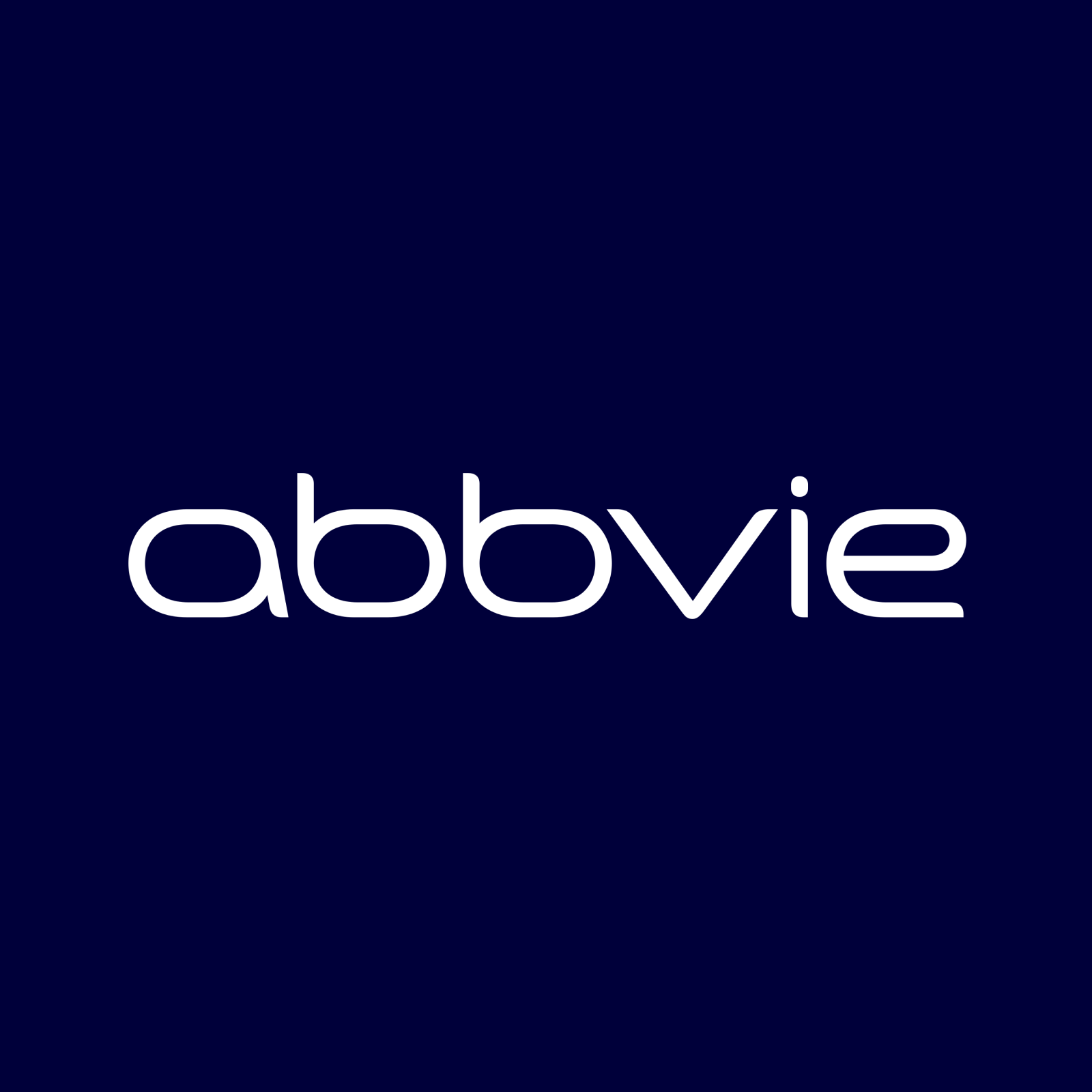# Abbvie Inc (NYSE:ABBV)

152.34 USD +2.89 USD ( +1.93% )Abbvie Inc
NYSE:ABBV

## DCF Value

Estimated DCF Value of oneABBV stock under the base case scenario is 125.4 USD. Compared to the current market price of 152.34 USD, the stock is Overvalued by 18%.

Estimated DCF Value of one NYSE:ABBV stock is 125.4 USD. Compared to the current market price of 152.34 USD, the stock is Overvalued by 18% .

DCF valuation is one of two methods of placing a monetary value on a company; the other is Relative Valuation method. We use a combination of these two methods to calculate the Intrinsic Value of stock as accurately as possible.

Discount Rate
8.52%
Terminal Growth
0%
Growth Period
5 Years
Discount Rate
8.52%
Terminal Growth
0%
Growth Period
5 Years

You can change any inputs, such as future revenue, using Present Value Calculation block.

DCF Valuation FAQ:
ABBV DCF Value
Base Case
125.4 USD
Overvaluation 18%
DCF Value
PriceAbbvie Inc Competitors:
DCF Valuation
FENC
Fennec Pharmaceuticals Inc
CELC
Celcuity Inc
4883
Modalis Therapeutics Corp
AUPH
Aurinia Pharmaceuticals Inc
CIR
Circassia Group PLC
TGTX
TG Therapeutics Inc
BTAI
BioXcel Therapeutics Inc

## DCF Value Calculation

### Capital Structure From Present Value to DCF ValuePresent Value 222B USD Equity Value 222B USD / Shares Outstanding 1.8B ABBV DCF Value 125.4 USD
Overvalued by 18%

To view the process of calculating the Present Value of Abbvie Inc' future free cash flow, see the Present Value Calculation block.

## Present Value Calculation

### Discounted Cash Flow Model Present Value of Free Cash FlowRotate your device for better experience.
Currency: USD

To view the process of deriving the DCF Value of one share from the estimated Present Value, see the DCF Value Calculation block.

## Sensitivity Analysis

### Sensitivity Analysis DCF Value Sensitivity AnalysisAnalyze the possible values of ABBV stock DCF Value given various model inputs such as Revenue Growth, Operating Margin, and Discount Rate.

Similar Stocks

## ABBV Stock DCF Valuation FAQ

What is the DCF value of one ABBV stock?

Estimated DCF Value of oneABBV stock under the base case scenario is 125.4 USD. Compared to the current market price of 152.34 USD, the stock is Overvalued by 18%.

The true DCF Value lies somewhere between the worst-case and best-case scenario values. This is because the future is not predetermined, and the stock's DCF Value is based almost entirely on the future of the company. Knowing the full range of possible stock intrinsic values gives a complete picture of the investment risks and opportunities.

How was the DCF Value calculated?

1. Present Value Calculation. Using the DCF Operating Model we projectAbbvie Inc's future free cash flow and discount it at a selected discount rate to calculate its Present Value (222B USD).

2. DCF Value Calculation. We use the company's capital structure to calculate the total Equity Value based on the previously computed Present Value of the free cash flow. Dividing the Equity Value by the number of shares outstanding gives us the DCF Value of 125.4 USD per oneABBV share.